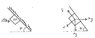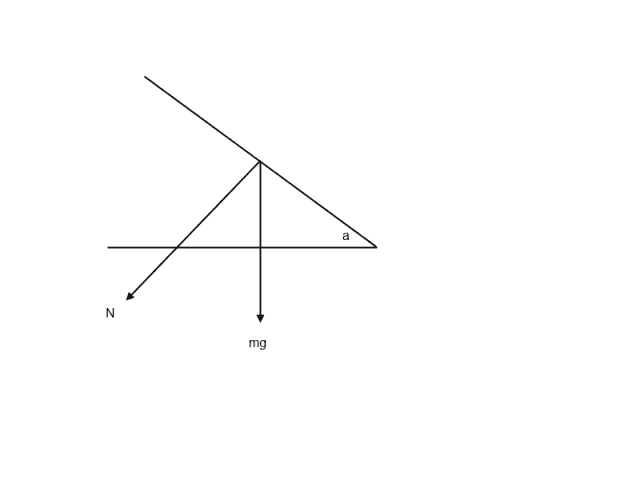# Equivalent angles and Trig Fucntions

TheCammen

## Homework Statement

A block with mass M is held statically on an overhang by a force Mg applied horizontally and the force of friction on the overhang. What are the normal and frictional forces? For what angles θ does the block remain at rest?

## The Attempt at a Solution

In the picture I've drawn out the forces acting on the block. I understand the ideas behind static equilibrium. What I have trouble with is understanding which trig functions to use when comparing the forces and how to identify which angles are equivalent to the angle θ in the problem. Can anyone explain the thought process behind determining these things?

#### Attachments

•Block Overhang.jpg
13.6 KB · Views: 459

Homework Helper
To get the forces N and Ff, you will have to split the weight into two components, one perpendicular to the plane and one parallel to the plane. If we redraw the two forces like this: (reducing the block to a small point)The angle formed by the line mg and the horizontal is 90 degrees and the angle formed by the normal of the plane and the plane itself is?

When you get that you can easily see where the angle a will be in relation to N and mg.

Draw in the 90 degree angles one at a time for each triangle.

azizlwl
The force that keep the block from falling is a component of frictional force.
FfSinθ=mg